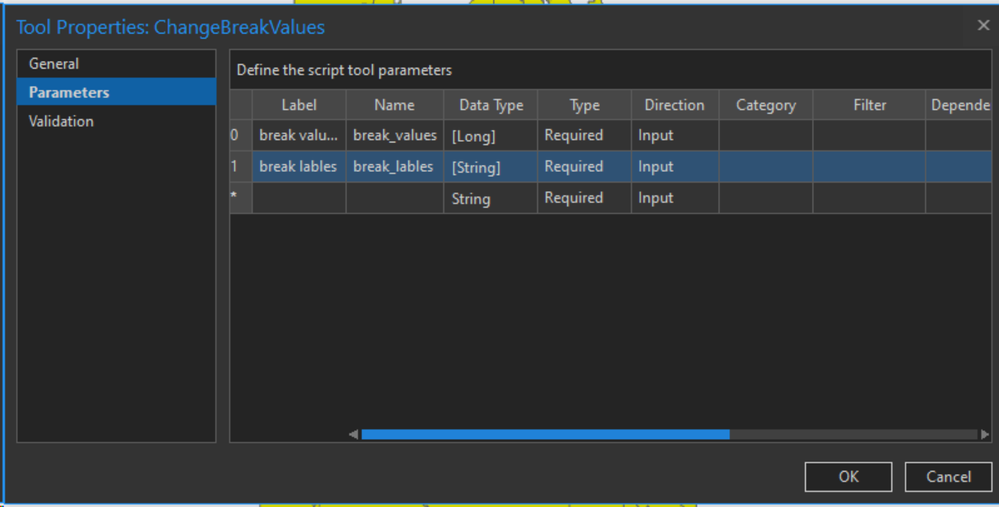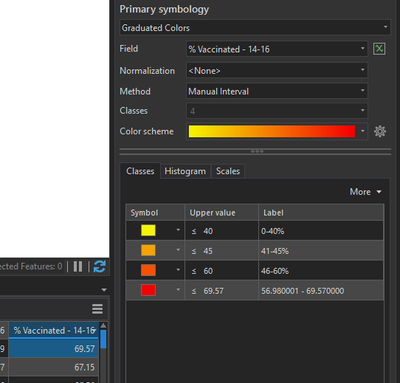# Custom script tool parameters will not accept decimals

347
8
10-18-2021 12:33 PMbyMVP Regular ContributorI have a script tool that sets a layer's break values and their labels. The user will also need to enter decimals as values. I can't seem to get the tool to do that. Here I have the break values set to accept a Long data type. I've also tried a double, and a string.

The break values entered into the tool are forced to be integers in the script.

``````#class break values
classbreakvalues = sys.argv #break value parameter entered here
breakvalues_list = classbreakvalues.split(";")
new_breakvalues_list = []
for brkvalues in breakvalues_list:
newbreak = int(brkvalues) # integer value
new_breakvalues_list.append(newbreak)
classBreakValues = new_breakvalues_list``````

If I run the following, the tool won't accept a decimal value.I get this error, which I understand to mean you can't have an string as an integer. But I don't see how that could be.

``ValueError: invalid literal for int() with base 10: '70.5'``

Tags (5)
1 Solution

Accepted Solutionsby
Regular Contributor

The int type can't have decimals so you need to use a float conversion if you want to persist the decimal values.

``newbreak = float(brkvalues) # integer value``

You can cast to float first then int, but it will truncate your number.

val = int(float(brkvalues))

8 RepliesbyMVP Esteemed Contributor
``classbreakvalues.split(";")``

suggests that the values are being returned as a semicolon delimited string.  If that is the cast them to a float when you get them.

``````vals = "40;45;70.5;100"

v = vals.split(";")

v0 = [float(i) for i in v]

v
['40', '45', '70.5', '100']

v0
[40.0, 45.0, 70.5, 100.0]``````

... sort of retired...by
Regular Contributor

The int type can't have decimals so you need to use a float conversion if you want to persist the decimal values.

``newbreak = float(brkvalues) # integer value``

You can cast to float first then int, but it will truncate your number.

val = int(float(brkvalues))byMVP Regular Contributor

``newbreak = [float(i) for i in brkvalues]``

@DanPattersonIf I use that it gives me: TypeError: int() argument must be a string, a bytes-like object or a number, not 'list'.

@JeffKI used float(brkvalues) and set the tool Data Type to Double. The tool ran with no error, but the value I set to a decimal was not changed in the symbology. And yes, I've noticed newbreak = int(float(brkvalues)) doesn't show the decimal places in the symbology either.byMVP Esteemed Contributor

you have to int a float not a string, hence int(float("1.1")), but int("1") is ok (refer to type conversion docs in python)

... sort of retired...byMVP Regular Contributor

Using newbreak = float(brkvalues) also does something with the highest value in a particular field. I set the last upper break value to be 100 in the tool parameters before I ran it. But, for some reason its grabbing the highest value in a particular layer's table and setting it as the last upper value in the symbology. Here, for example, 69.57 is the highest value in the % Vaccinated - 14-16 field. It ignores my label and it shows a decimal?byMVP Regular ContributorAll three of the following arrangements in the code are successful when running them from the script tool. But, not one of them change the break value to a decimal in the layer's symbology. This leads me to believe something is up with the tool.

``````#Results in neither decimal in layer symbology nor print statement
for brkvalues in breakvalues_list:
newbreak = int(float(brkvalues))

#prints these values
#break value: 40
#break value: 45
#break value: 60
#break value: 100

#No decimal in layer symbology, but decimal in print statement
for brkvalues in breakvalues_list:
newbreak = float(brkvalues)

#prints these values
#break value: 40
#break value: 45
#break value: 60.9
#break value: 100

#No decimal in layer symbology, but decimal in print statement
from decimal import Decimal
for brkvalues in breakvalues_list:
newbreak = Decimal(brkvalues)

#prints these values
#break value: 40
#break value: 45
#break value: 60.9
#break value: 100``````byMVP Regular Contributor

OK, I was too focused on one part of the script. The snippet I had posted above was the section of code that takes the users input and puts it into a variable. Then there is the other part of the script the assigns that input to the upperBound element of the symbology. This is what I needed to convert to a float! Thanks for your answers.

``````import arcpy, sys

aprx = arcpy.mp.ArcGISProject('CURRENT')

#make sure fields in list are in same order as maps in project
fields =  ['VaccPercentageTotPop', 'VaccPercentage11to14', 'VaccPercentage0to18', 'VaccPercentage12to18',
'VaccPercentage14to16', 'VaccPercentage16to18', 'VaccPercentage19to64', 'VaccPercentage65Plus']

c = 0 #use counter to give a number to each map in for loop
for map in aprx.listMaps():
layers = map.listLayers()
for layer in layers:
sym = layer.symbology
#Reset renderer
sym.updateRenderer('SimpleRenderer')

if hasattr(sym, 'renderer') and layer.name.startswith('BaseLayer'):
Renderer = sym.renderer
Renderer.classificationField = fields[c]
#class break values
classbreakvalues = arcpy.GetParameterAsText(0)
breakvalues_list = classbreakvalues.split(";")
new_breakvalues_list = []
for brkvalues in breakvalues_list:
newbreak = brkvalues
new_breakvalues_list.append(newbreak)
classBreakValues = new_breakvalues_list
#class break labels
classbreaklabels = arcpy.GetParameterAsText(1)
breaklabels_list = classbreaklabels.split(";")
classBreakLabels = breaklabels_list

# Run the Renderer.breakCount function to use the classBreakValues
# array parameters as the values, and create a counter.
Renderer.breakCount = len(classBreakValues)

i = 0
for brk in Renderer.classBreaks:
brk.upperBound = float(classBreakValues[i]) #<-- Convert this to float
brk.label = classBreakLabels[i]
i+=1

layer.symbology = sym
c+=1
``````byMVP Regular Contributor

Interestingly, all I had to do was change arcpy.GetParameterAsText() to arcpy.GetParameter(). Then I didn't even have to bother with later changing the value to a float.